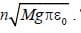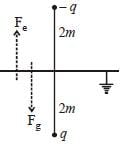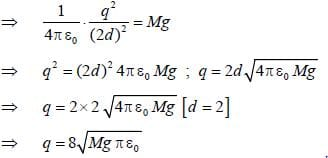A charge particle of mass M is placed below t...

### Related TestA charge particle of mass M is placed below the grounded infinite conducting plate lies in xy-plane. If the distance between the charge particle and plate is 2m then the magnitude of charge particle so that it remains stationary isThe value of n is _________________ (answer should be integer)ShakuntlaLet the magnitude of charge particle is q.
The cliarge particle will remain stationary ifView courses related to this question Explore GATE courses
 Explore GATE coursesView courses related to this question1 Crore+ students have signed up on EduRev. Have you?

• ### A point charge is placed between two semi- infinite conducting plates which... more(Scan QR code)